## 11.5.4 Gaining Information Without Sensors

For some problems, it is remarkable that uncertainty may be reduced without even using sensors. Recall Example 11.17. This is counterintuitive because it seems that information regarding the state can only be gained from sensing. It is possible, however, to also gain information from the knowledge that some actions have been executed and the effect that should have in terms of the state transitions. The example presented in this section is inspired by work on sensorless manipulation planning [321,396], which is covered in more detail in Section 12.5.2. This topic underscores the advantages of reasoning in terms of an I-space, as opposed to requiring that accurate state estimates can be made.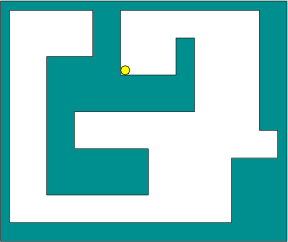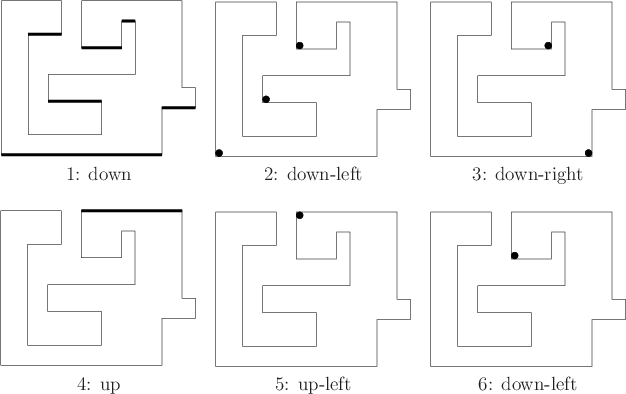Example 11..24 (Tray Tilting)   The state space,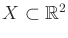, indicates the position of a ball that rolls on a flat surface, as shown Figure 11.28. The ball is confined to roll within the polygonal region shown in the figure. It can be imagined that the ball rolls in a tray on which several barriers have been glued to confine its motion (try this experiment at home!). If the tray is tilted, it is assumed that the ball rolls in a direction induced by gravity (in the same way that a ball rolls to the bottom of a pinball machine).

The tilt of the tray is considered as an action that can be chosen by the robot. It is assumed that the initial position of the ball (initial state) is unknown and there are no sensors that can be used to estimate the state. The task is to find some tilting motions that are guaranteed to place the ball in the position shown in Figure 11.28, regardless of its initial position.

The problem could be modeled with continuous time, but this complicates the design. If the tray is tilted in a particular orientation, it is assumed that the ball rolls in a direction, possibly following the boundary, until it comes to rest. This can be considered as a discrete-stage transition: The ball is in some rest state, a tilt action is applied, and a then it enters another rest state. Thus, a discrete-stage state transition equation,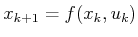, is used.

To describe the tilting actions, we can formally pick directions for the upward normal vector to the tray from the upper half of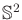; however, this can be reduced to a one-dimensional set because the steepness of the tilt is not important, as long as the ball rolls to its new equilibrium state. Therefore, the set of actions can be considered as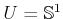, in which a direction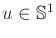indicates the direction that the ball rolls due to gravity. Before any action is applied, it is assumed that the tray is initially level (its normal is parallel to the direction of gravity). In practice, one should be more careful and model the motion of the tray between a pair of actions; this is neglected here because the example is only for illustrative purposes. This extra level of detail could be achieved by introducing new state variables that indicate the orientation of the tray or by using continuous-time actions. In the latter case, the action is essentially providing the needed state information, which means that the action function would have to be continuous. Here it is simply assumed that a sequence of actions from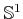is applied.

The initial condition is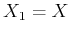and the history I-state is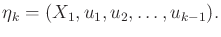(11.74)

Since there are no observations, the path through the I-space is predictable. Therefore, a plan,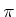, is simply an action sequence,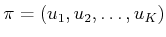, for any desired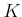.

It is surprisingly simple to solve this task by reasoning in terms of nondeterministic I-states, each of which corresponds to a set of possible locations for the ball. A sequence of six actions, as shown in Figure 11.29, is sufficient to guarantee that the ball will come to rest at the goal position, regardless of its initial position.Steven M LaValle 2020-08-14